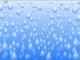# Unresolved Detail In Plotted Equations

Sometimes the calculator detects that an equation is too complicated to plot perfectly in a reasonable amount of time. When this happens, the equation is plotted at lower resolution.

267 out of 297 found this helpful
Have more questions? Submit a request

•Monkey D Luffy

\cos \left(xy^2\right)=\arctan \left(\sin \left(3yx\right)\right)

interesting...

•Monkey D Luffy

\tan \left(yx^2\right)=\cos \left(x^{10}-2y^x\right)

•MJ Kim

y^{x!}=x

•Bombz z

x^2+y^2=\cos \left(x^2y^2\right) apperantly...

•InfernoGaming1

What is the money sign for when doing functions?

•InfernoGaming1

Also Try

$y>= |sin x| |cos y| |tan x| |csc y| |sec x| |cot y|$

$y>= – |sin x| |cos y| |tan x| |csc y| |sec x| |cot y|$

•Bombz z

\left(\tan x\right)\left(\tan y\right)=a

change the a value all you want

•Tiyer283

Another equation is : y=yx^yx

•Kang Oedin
•Monkey D Luffy

world of blocks

\operatorname{round}\left(\cos \left(y^2\right)\right)=\operatorname{round}\left(\sin \left(x\right)\right)

or

\operatorname{round}\left(\cos \left(y^2\right)\right)=\operatorname{round}\left(\sin \left(x^2\right)\right)

•Alex Post

x squared+y squared+= round(x squared+y squared). A beautiful one. So is x*y=round(x*y) The round command can be used a lot to break this.

•Mr A.

Crazy but cool at the same time -

y=\sin \left(x-y^2\right)\left(y-x^2\right)•Jhuber
•Jhuber

navagate the green dot up without hitting the red lines https://www.desmos.com/calculator/c4hnr3hyup

•Jhuber

also the lines that makes a heart doesn't count

•Jhuber

mod(cos(x),sin(x))

•Jhuber

this one has limits and what I call flareons https://www.desmos.com/calculator/gbhhofzbrv

•Cobalt

Some cool ones:

\tan \left(\sqrt{\left|x\right|}\right)=\tan \left(\sqrt{\left|y\right|}\right)

\tan \left(x!\cdot y!\right)^2=1    <---This one lags alot but is very cool

\tan \left(yx\right)=-x

\sqrt{\left(x-y\right)}=\frac{\tan \left(x^2\right)}{\tan \left(y\right)}   <---This one looks like a footprint

\tan \left(x+y\right)^{\tan \left(e\right)}=\frac{\tan \left(y-x\right)}{2}   <---This one looks like the ocean

\tan \left(\operatorname{floor}\left(x\right)\right)\le \tan \left(\frac{\operatorname{ceil}\left(y\right)}{\operatorname{sign}\left(y\right)}\right)    <---This one is pixelated

\tan \left(xyxyxyxyxyxyxyxyxyxyxyxyxy\right)=1

\tan \left(x^2+y^2\right)=\sin \left(x\right)

\frac{\csc \left(x\right)}{\operatorname{floor}\left(y\right)}=\frac{\sqrt{\frac{xy}{2}}}{\operatorname{ceil}\left(x\right)}

\frac{\tan x^2}{\tan y^2}>\frac{\tan y^2}{\tan x^2}  <---True chaos

\left|\sin \left(x^2-y^2\right)\right|=\sin \left(x+y\right)+\cos \left(x\cdot y\right)

x^2\cos (x)=y\tan (y)

\sin \left(x^2+y^2\right)=\cos \left(x\cdot y\right)   <---This one looks like an atom

e^{\sin \left(x\right)+\cos \left(y\right)}=\sin \left(e^{x+y}\right)   <---My favorite

\sinh x^2\ge \operatorname{floor}\left(6y\right)

\frac{\sin x}{\operatorname{ceil}\left(y\right)}=\frac{\sqrt{\frac{\sin y}{\operatorname{floor}\left(x\right)}}}{\sqrt{\frac{\sin x}{\operatorname{floor}\left(y\right)}}}

\frac{\tan x^2}{\tan y^2}=\frac{\tan y^2}{\tan x^2}

\sin \left(7x\right)^2=\left(\sin \left(y\right)^2\cdot \tan \left(x\right)^2\right)

•Evan Bailey

Not really that cool, but still has unresolved detail:

Copy into Desmos:

y=\sqrt{3x+100\cos \left(y^2\right)}

Standard Formatting:

y=sqrt(3x+100cos(y^2))

•Cobalt

Best way to crash your CPU:

tan(x!*y!)=1

•Wul531826

try tan(x!y!)^(x!y!)=1

•Jhuber

https://www.desmos.com/calculator/28kaxgqagi try to figure out how to make a code and look for squares.

https://www.desmos.com/calculator/hbrjlel3re enjoy my fake galixcy

•Jhuber
•NathoMIL1416- Coding Tutorials Minecraft and More!

For some PURE INSANITY: sin(cos(tan(x^2)))=sin(cos(tan(y^2)

For ALL the circles possible: sin(x^2)=cos(y^2)

•Jet Taylor

Go to my other post. Please.

Edited by Jet Taylor
•Jet Taylor

0=\sin xy

Little function, big impact.

*POW*

0=\sin \left(x\right)\sin \left(y\right)

My computer ran slower.

1=a_1\sinx\cosx+a_2\sinx\cosy+a_3\siny\cosx+a_4\siny\cosy

I got drowned by the ocean.

Edited by Jet Taylor
•try  (x^2-y^2)^2+(xy)^2=(x^2+y^2)^2

•菅原悠人

This draws countless ∞.

sin(x)!=cos(y)

•Jhuber

Press play for crasyness

•Liam Watson

y=x^{y^x}-y^{x^y}